P-value functions: Tutorial using the pvaluefunctions package

Accompanying paper

We published an accompanying paper to illustrate the use of p-value functions:

Infanger D, Schmidt-Trucksäss A. (2019): P value functions: An underused method to present research results and to promote quantitative reasoning. Statistics in Medicine, 38: 4189-4197. doi: 10.1002/sim.8293.

Recreation of the graphics in the paper

The code and instructions to reproduce all graphics in our paper can be found in the following GitHub repository: https://github.com/DInfanger/pvalue_functions

Overview

This vignette shows how to use the pvaluefunctions package wich contains an R function to create graphics of p-value functions, confidence distributions, confidence densities, or the Surprisal value (S-value) (Greenland 2019).

Installation

Install (install.packages("pvaluefunctions")) and load the package from CRAN.

library(pvaluefunctions)

Dependencies

The function depends on the following R packages:

Usage

There is only one function needed to create the plots: conf_dist(). The function has the following arguments:

• estimate: Numerical vector containing the estimate(s).
• n: Numerical vector containing the sample size(s). Required for correlations, variances, proportions and differences between proportions. Must be equal the number of estimates.
• df: Numerical vector containing the degrees of freedom. Required for statistics based on the t-distribution (e.g. linear regression) and t-tests. Must be equal the number of estimates.
• stderr: Numerical vector containing the standard error(s) of the estimate(s). Required for statistics based on the t-distribution (e.g. linear regression) and the normal distribution (e.g. logistic regression). Must be equal the number of estimate(s).
• tstat: Numerical vector containing the t-statistic(s). Required for t-tests (means and mean differences). Must be equal the number of estimates.
• type: String indicating the type of the estimate. Must be one of the following: ttest, linreg, gammareg, general_t, logreg, poisreg, coxreg, general_z, pearson, spearman, kendall, var, prop, propdiff.
• plot_type: String indicating the type of plot. Must be one of the following: cdf (confidence distribution), pdf (confidence density), p_val (p-value function, the default), s_val (Surprisal). For differences between independent proportions, only p-value functions and Surprisal value functions are available.
• n_values (optional): Integer indicating the number of points that are used to generate the graphics. The higher this number, the higher the computation time and resolution.
• est_names (optional): String vector indicating the names of the estimate(s). Must be equal the number of estimates.
• conf_level (optional): Numerical vector indicating the confidence level(s). Bust be between 0 and 1.
• null_values (optional): Numerical vector indicating the null value(s) in the plot on the untransformed scale. For example: If you want to plot odds ratios from logistic regressions, the null_values have to be given on the log-odds scale. If x limits are specified with xlim, all null values outside of the specified x limits are ignored for plotting and a message is printed.
• trans (optional): String indicating the transformation function that will be applied to the estimates and confidence curves. For example: "exp" for an exponential transformation of the log-odds in logistic regression.
• alternative: String indicating if the confidence level(s) are two-sided or one-sided. Must be one of the following: two_sided, one_sided.
• log_yaxis: Logical. Indicating if a portion of the y-axis should be displayed on the logarithmic scale.
• cut_logyaxis: Numerical value indicating the threshold below which the y-axis will be displayed logarithmically. Must lie between 0 and 1.
• xlim (optional) Optional numerical vector of length 2 (x1, x2) indicating the limits of the x-axis on the untransformed scale. For example: If you want to plot p-value functions for odds ratios from logistic regressions, the limits have to be given on the log-odds scale. Note that x1 > x2 is allowed but then x2 will be the left limit and x1 the right limit (i.e. the limits are sorted before plotting). Null values (specified in null_values) that are outside of the specified limits are ignored and a message is printed.
• together: Logical. Indicating if graphics for multiple estimates should be displayed together or on separate plots.
• plot_legend Logical. Indicating if a legend should be plotted if multiple curves are plotted together with different colors (i.e. together = TRUE and same_color = FALSE).
• same_color: Logical. Indicating if curves should be distinguished using colors if they are plotted together (i.e. together = TRUE). Setting this to FALSE also disables the default behavior that the two halves of the curves are plotted in different colors for a one-sided alternative.
• col String indicating the colour of the curves. Only relevant for single curves, multiple curves not plotted together (i.e. together = FALSE) and multiple curves plotted together but with the option same_color set to TRUE.
• nrow: (optional) Integer greater than 0 indicating the number of rows when together = FALSE is specified for multiple estimates. Used in facet_wrap in ggplot2.
• ncol: (optional) Integer greater than 0 indicating the number of columns when together = FALSE is specified for multiple estimates. Used in facet_wrap in ggplot2.
• plot_p_limit: Numerical value indicating the lower limit of the y-axis. Must be greater than 0 for a logarithmic scale (i.e. log_yaxis = TRUE). The default is to omit plotting p-values smaller than 1 - 0.999 = 0.001.
• plot_counternull: Logical. Indicating if the counternull should be plotted as a point. Only available for -value functions and s-value functions. Counternull values that are outside of the plotted functions are not shown.
• title (optional): String containing a title for the plot.
• xlab (optional): String indicating the label of the x-axis.
• ylab (optional): String containing a title for the primary (left) y-axis.
• ylab_sec (optional): String containing a title for the secondary (right) y-axis.
• inverted Logical. Indicating the orientation of the P-value function (p_val), S-value function (s_val) and confidence distribution (cdf). By default (i.e. inverted = FALSE) small P-values are plotted at the bottom and large ones at the top. By setting inverted = TRUE, the y-axis is invertedd. Ignored for confidence densities.
• x_scale String indicating the scaling of the x-axis. The default is to scale the x-axis logarithmically if the transformation specified in trans is “exp” (exponential) and linearly otherwise. The option linear (can be abbreviated) forces a linear scaling and the option logarithm (can be abbreviated) forces a logarithmic scaling.
• plot Logical. Should a plot be created (TRUE, the default) or not (FALSE). FALSE can be useful if users want to create their own plots. If FALSE, no ggplot2 object is returned.

Required arguments for different estimate types

• t-tests: estimate, df, tstat.
• Linear regression, Gamma regression, general estimates with inference based on the t-distribution: estimate, df, stderr.
• Logistic regression, Poisson regression, Cox regression, general estimates with inference based on the normal distribution: estimate, stderr.
• Correlation coefficients (Pearson, Spearman, Kendall), proportions, difference between proportions, variances: estimate, n.

Returned values

The main function conf_dist() returns five objects in a list:

• res_frame: A data frame containing the values used to construct the plot. It contains the following variables: values contain the values of the effect (e.g. mean difference, odds ratio etc.), conf_dist the values for the confidence distribution, conf_dens the values for the confidence density, p_two the values for the two-sided p-value function, p_one the values for the one-sided p-value function, s_val the S-value (surprisal) of the two-sided p-values, variable the name of the estimate, hypothesis indicating the alternative hypothesis if one-sided p-value functions were specified and counternull containing the counternull values.
• conf_frame: A data frame containing the confidence intervals for the specified confidence levels for all estimates.
• counternull_frame: A data frame containing the counternull values for the specified null values (see Rosenthal & Rubin (1994) for more information about the counternull).
• point_est: A data frame containing the point estimates for all estimates. The point estimates correspond to the mean, median or mode of the confidence density (see Xie & Singh (2013) for more information). Estimates are produced using numerical procedures: Increase the number of points n_values for higher numerical precision.
• aucc_frame: A data frame containing the estimated AUCC (area under the confidence curve). The AUCC is a measures of precision with higher values indicating a poorer precision compared to smaller values (see Berrar (2017)). It also provides the proportion of the AUCC that lies above the null value(s), if they are provided.
• plot: A ggplot2 plot object (only returned if the option plot = TRUE was specified).

Examples

Two-sample t-test with unequal variances (Welch-Test)

#-----------------------------------------------------------------------------
# T-Test
#-----------------------------------------------------------------------------

with(sleep, mean(extra[group == 1])) - with(sleep, mean(extra[group == 2]))
#>  -1.58
t.test(extra ~ group, data = sleep, var.equal = FALSE)
#>
#>  Welch Two Sample t-test
#>
#> data:  extra by group
#> t = -1.8608, df = 17.776, p-value = 0.07939
#> alternative hypothesis: true difference in means between group 1 and group 2 is not equal to 0
#> 95 percent confidence interval:
#>  -3.3654832  0.2054832
#> sample estimates:
#> mean in group 1 mean in group 2
#>            0.75            2.33

#-----------------------------------------------------------------------------
# Create p-value function
#-----------------------------------------------------------------------------

res <- conf_dist(
estimate = c(-1.58)
, df = c(17.77647)
, tstat = c(-1.860813)
, type = "ttest"
, plot_type = "p_val"
, n_values = 1e4L
# , est_names = c("")
, conf_level = c(0.95, 0.90, 0.80)
, null_values = c(0)
, trans = "identity"
, alternative = "two_sided"
, log_yaxis = FALSE
, cut_logyaxis = 0.05
, xlab = "Mean difference (group 1 - group 2)"
, together = FALSE
, plot_p_limit = 1 - 0.999
, plot_counternull = TRUE
, x_scale = "line"
, plot = TRUE
)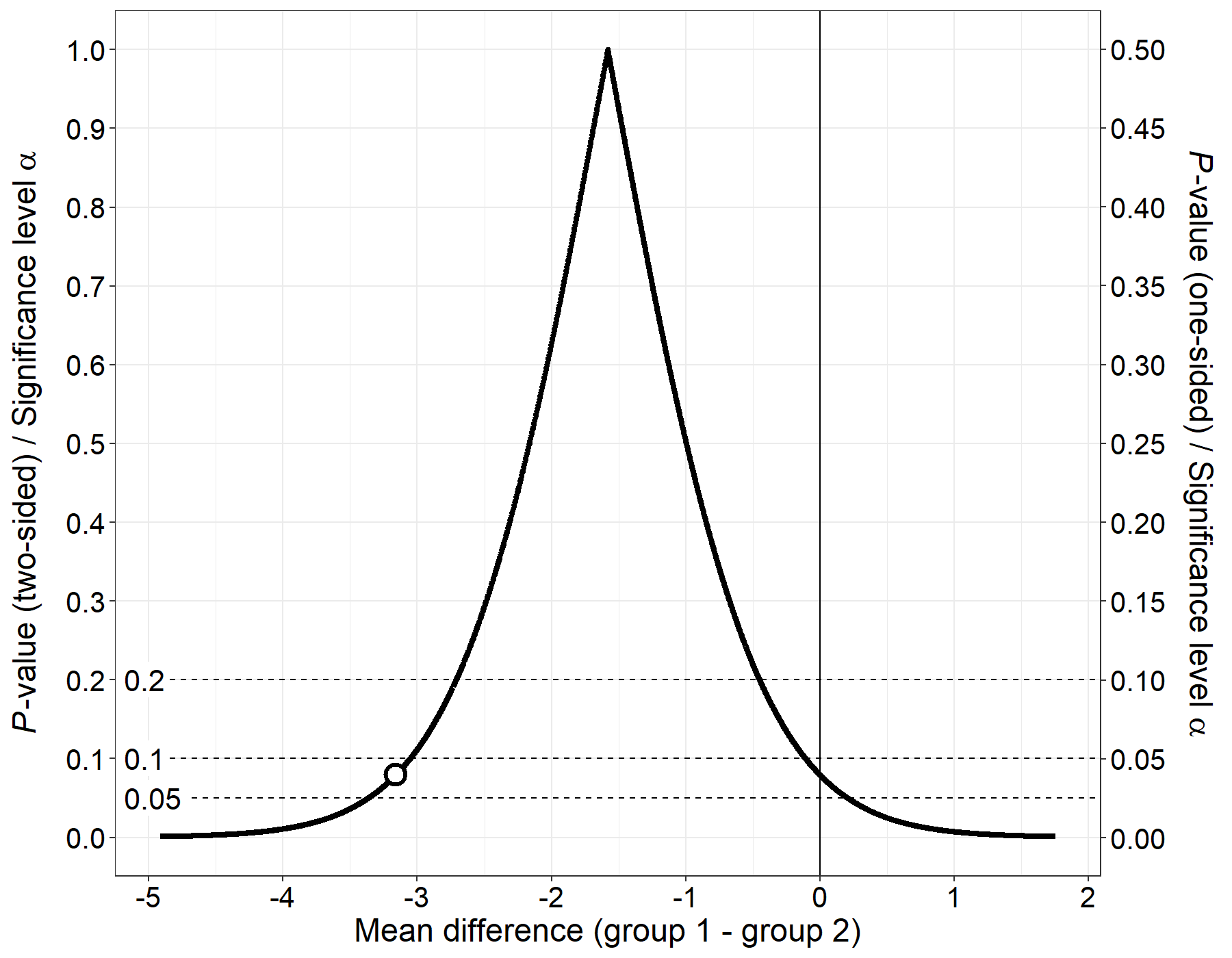Single coefficient from a linear regression model

P-value function

Because it’s difficult to see very small p-values in the graph, you can set the option log_yaxis = TRUE so that p-values (i.e. the y-axes) below the value set in cut_logyaxis will be plotted on a logarithmic scale. This will make it much easier to see small p-values but has the disadvantage of creating a “kink” in the p-value function which is a pure artifact and puts an undue emphasis on the specified cutoff.

#-----------------------------------------------------------------------------
# Model
#-----------------------------------------------------------------------------

mod <- lm(Infant.Mortality~Agriculture + Fertility + Examination, data = swiss)

summary(mod)
#>
#> Call:
#> lm(formula = Infant.Mortality ~ Agriculture + Fertility + Examination,
#>     data = swiss)
#>
#> Residuals:
#>     Min      1Q  Median      3Q     Max
#> -8.5375 -1.4021 -0.0066  1.7381  5.9150
#>
#> Coefficients:
#>             Estimate Std. Error t value Pr(>|t|)
#> (Intercept) 11.01896    4.47291   2.463  0.01784 *
#> Agriculture -0.02143    0.02394  -0.895  0.37569
#> Fertility    0.13115    0.04145   3.164  0.00285 **
#> Examination  0.04913    0.08351   0.588  0.55942
#> ---
#> Signif. codes:  0 '***' 0.001 '**' 0.01 '*' 0.05 '.' 0.1 ' ' 1
#>
#> Residual standard error: 2.645 on 43 degrees of freedom
#> Multiple R-squared:  0.2291, Adjusted R-squared:  0.1753
#> F-statistic:  4.26 on 3 and 43 DF,  p-value: 0.01014

#-----------------------------------------------------------------------------
# Create p-value function
#-----------------------------------------------------------------------------

res <- conf_dist(
estimate = c(-0.02143)
, df = c(43)
, stderr = (0.02394)
, type = "linreg"
, plot_type = "p_val"
, n_values = 1e4L
# , est_names = c("")
, conf_level = c(0.95, 0.90, 0.80)
, null_values = c(0)
, trans = "identity"
, alternative = "two_sided"
, log_yaxis = TRUE
, cut_logyaxis = 0.05
, xlab = "Coefficient Agriculture"
, xlim = c(-0.12, 0.065)
, together = FALSE
, plot_p_limit = 1 - 0.999
, plot_counternull = FALSE
, plot = TRUE
)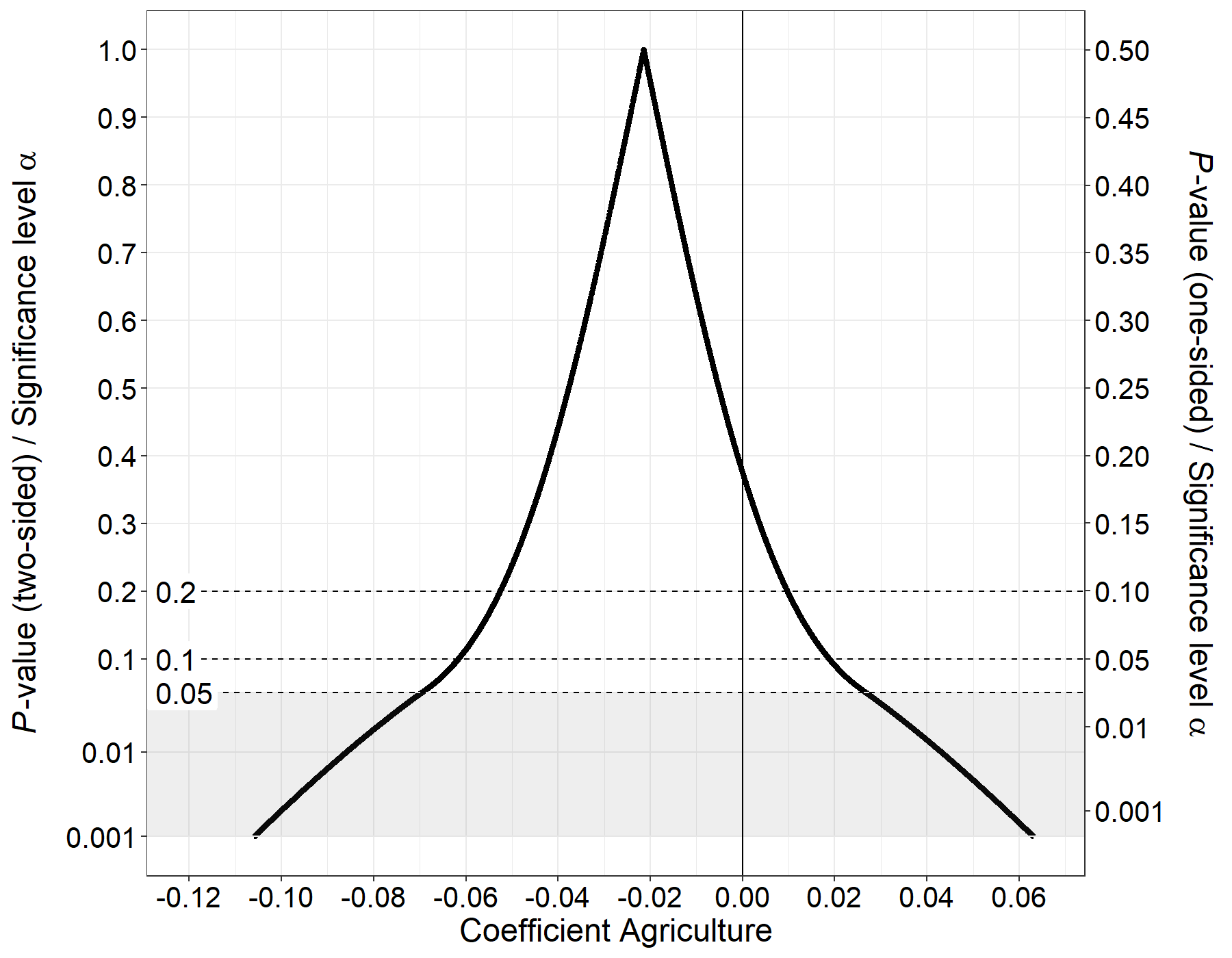Confidence distribution

res <- conf_dist(
estimate = c(-0.02143)
, df = c(43)
, stderr = (0.02394)
, type = "linreg"
, plot_type = "cdf"
, n_values = 1e4L
# , est_names = c("")
, conf_level = c(0.95, 0.90, 0.80)
, null_values = c(0)
, trans = "identity"
, alternative = "two_sided"
# , log_yaxis = TRUE
# , cut_logyaxis = 0.05
, xlab = "Coefficient Agriculture"
, xlim = c(-0.12, 0.065)
, together = FALSE
, col = "#08A9CF"
# , plot_p_limit = 1 - 0.999
, plot_counternull = FALSE
)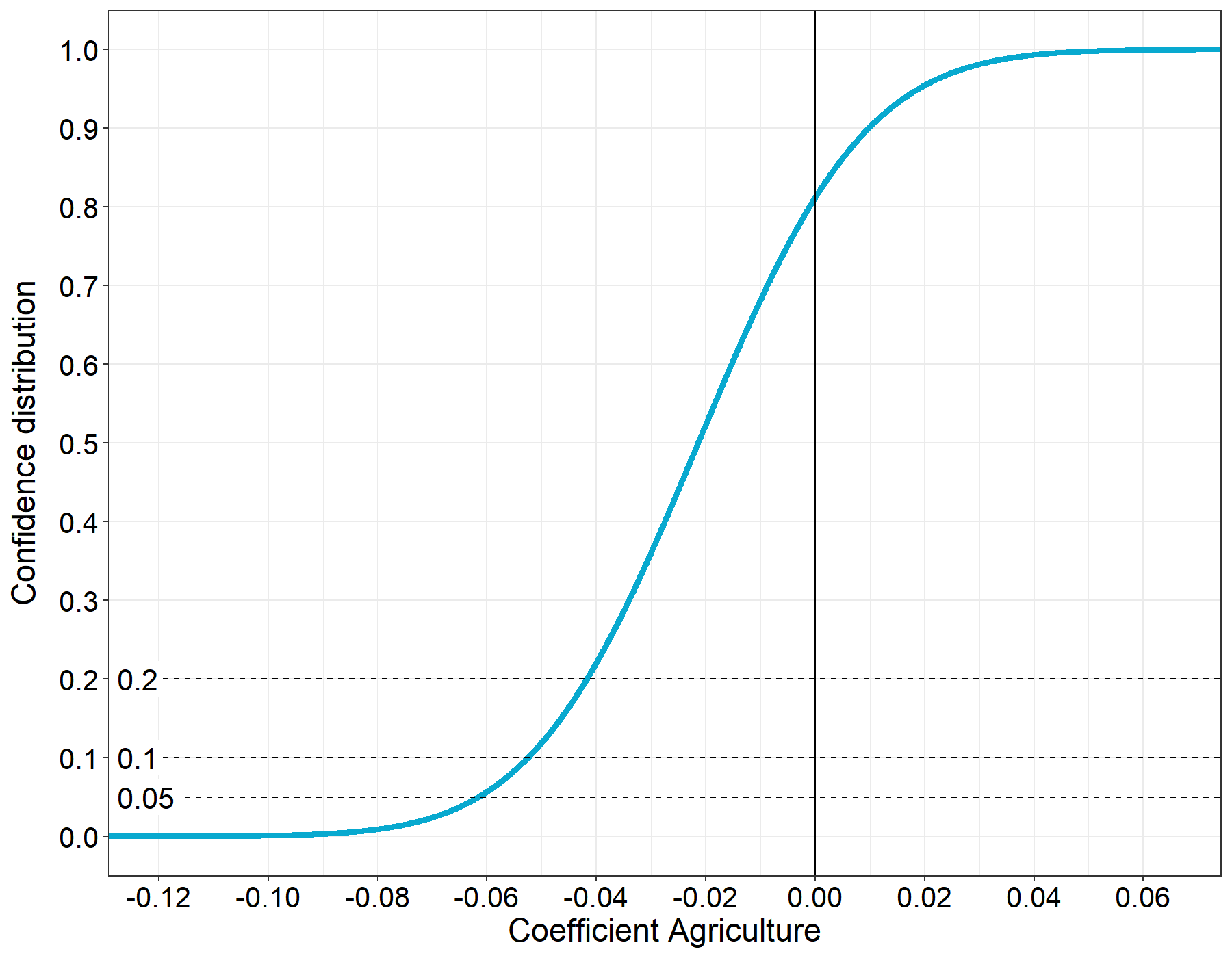The point where the confidence distribution is \(0.5\) is the median unbiased estimator (see Xie & Singh (2013) for a review and proofs).

Multiple coefficients from a linear regression model

P-value functions

res <- conf_dist(
estimate = c(0.13115, 0.04913)
, df = c(43, 43)
, stderr = c(0.04145, 0.08351)
, type = "linreg"
, plot_type = "p_val"
, n_values = 1e4L
, est_names = c("Fertility", "Examination")
, conf_level = c(0.95, 0.90, 0.80)
, null_values = c(0)
, trans = "identity"
, alternative = "two_sided"
, log_yaxis = FALSE
, cut_logyaxis = 0.05
, xlab = "Regression coefficients"
, together = TRUE
, same_color = FALSE
, plot_p_limit = 1 - 0.999
, plot_counternull = FALSE
, inverted = FALSE
)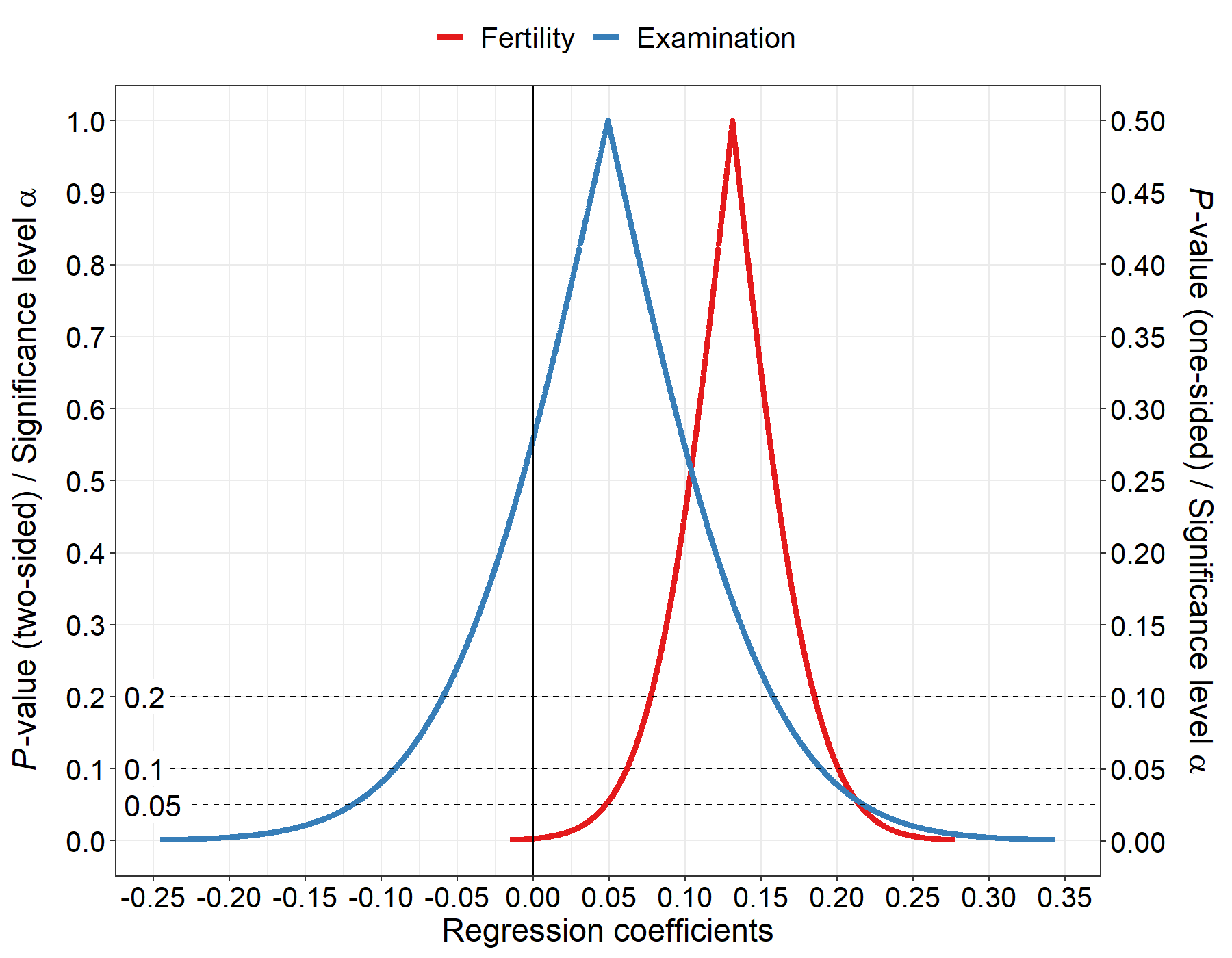Surprisal values

res <- conf_dist(
estimate = c(0.13115, 0.04913)
, df = c(43, 43)
, stderr = c(0.04145, 0.08351)
, type = "linreg"
, plot_type = "s_val"
, n_values = 1e4L
, est_names = c("Fertility", "Examination")
, conf_level = c(0.95, 0.90, 0.80)
, null_values = c(0)
, trans = "identity"
, alternative = "two_sided"
# , log_yaxis = TRUE
# , cut_logyaxis = 0.05
, xlab = "Regression coefficients"
, together = TRUE
, plot_p_limit = 1 - 0.999
, plot_counternull = TRUE
)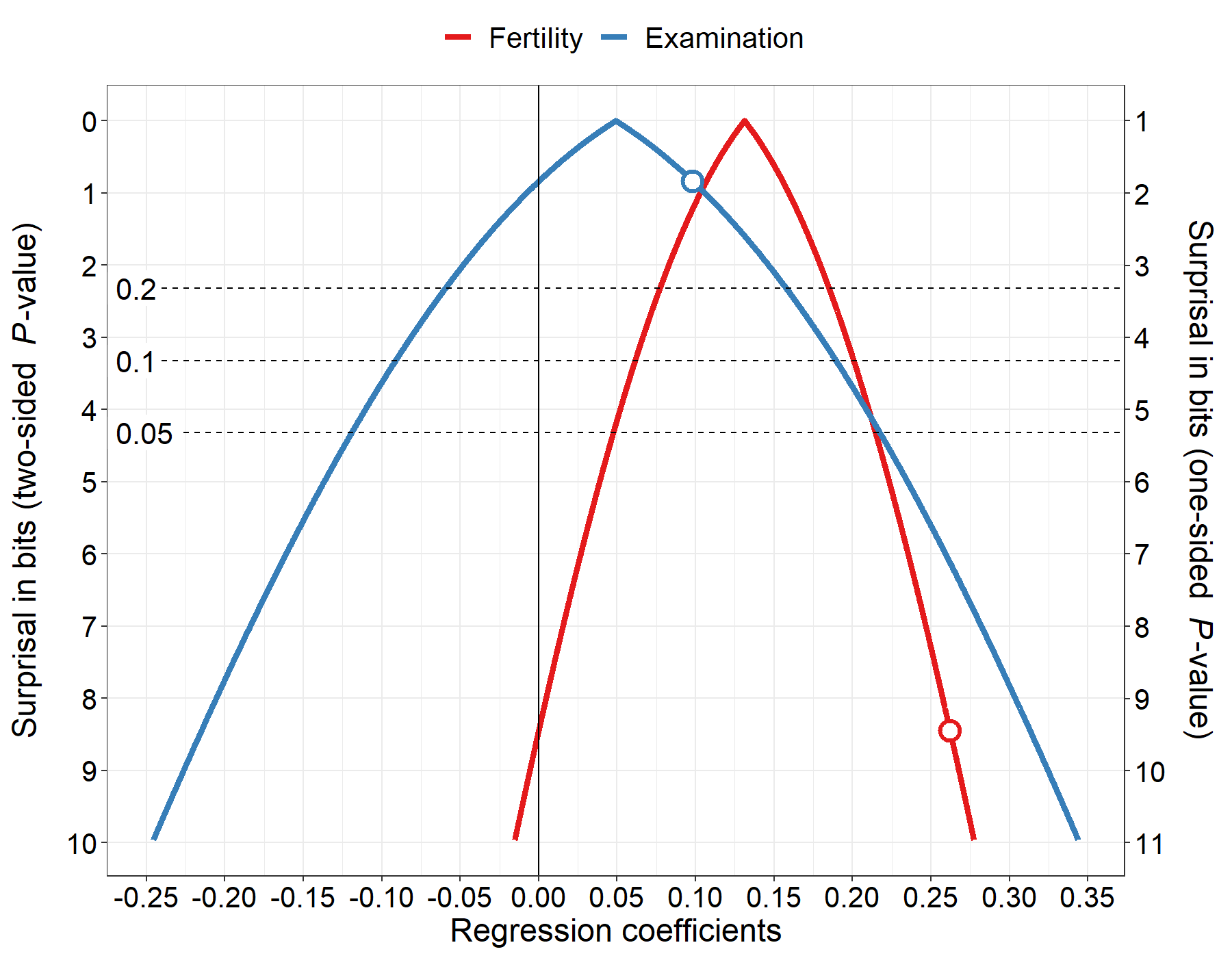Pearson correlation coefficient (one-sided)

As of version 1.6.2, the package uses the exact distribution for Pearson’s correlation coefficient as described in a preprint by Gunnar Taraldsen (2020). The calculations make use of the Gaussian hypergeometric function (\(_2F_1\)). It also makes the calculations slower, depending on the value in n_values.

#-----------------------------------------------------------------------------
# Calculate Pearson's correlation coefficient
#-----------------------------------------------------------------------------

cor.test(swiss\$Fertility, swiss\$Agriculture, alternative = "two.sided", method = "pearson")
#>
#>  Pearson's product-moment correlation
#>
#> data:  swiss\$Fertility and swiss\$Agriculture
#> t = 2.5316, df = 45, p-value = 0.01492
#> alternative hypothesis: true correlation is not equal to 0
#> 95 percent confidence interval:
#>  0.07334947 0.58130587
#> sample estimates:
#>       cor
#> 0.3530792

#-----------------------------------------------------------------------------
# Create p-value function
#-----------------------------------------------------------------------------

res <- conf_dist(
estimate = c(0.3530792)
, n = 47
, type = "pearson"
, plot_type = "p_val"
, n_values = 1e3L
# , est_names = c("")
, conf_level = c(0.95, 0.90, 0.80)
, null_values = c(0)
, trans = "identity"
, alternative = "one_sided"
, log_yaxis = FALSE
, cut_logyaxis = 0.05
, xlab = "Pearson correlation"
, together = TRUE
, plot_p_limit = 1 - 0.999
, plot_counternull = FALSE
)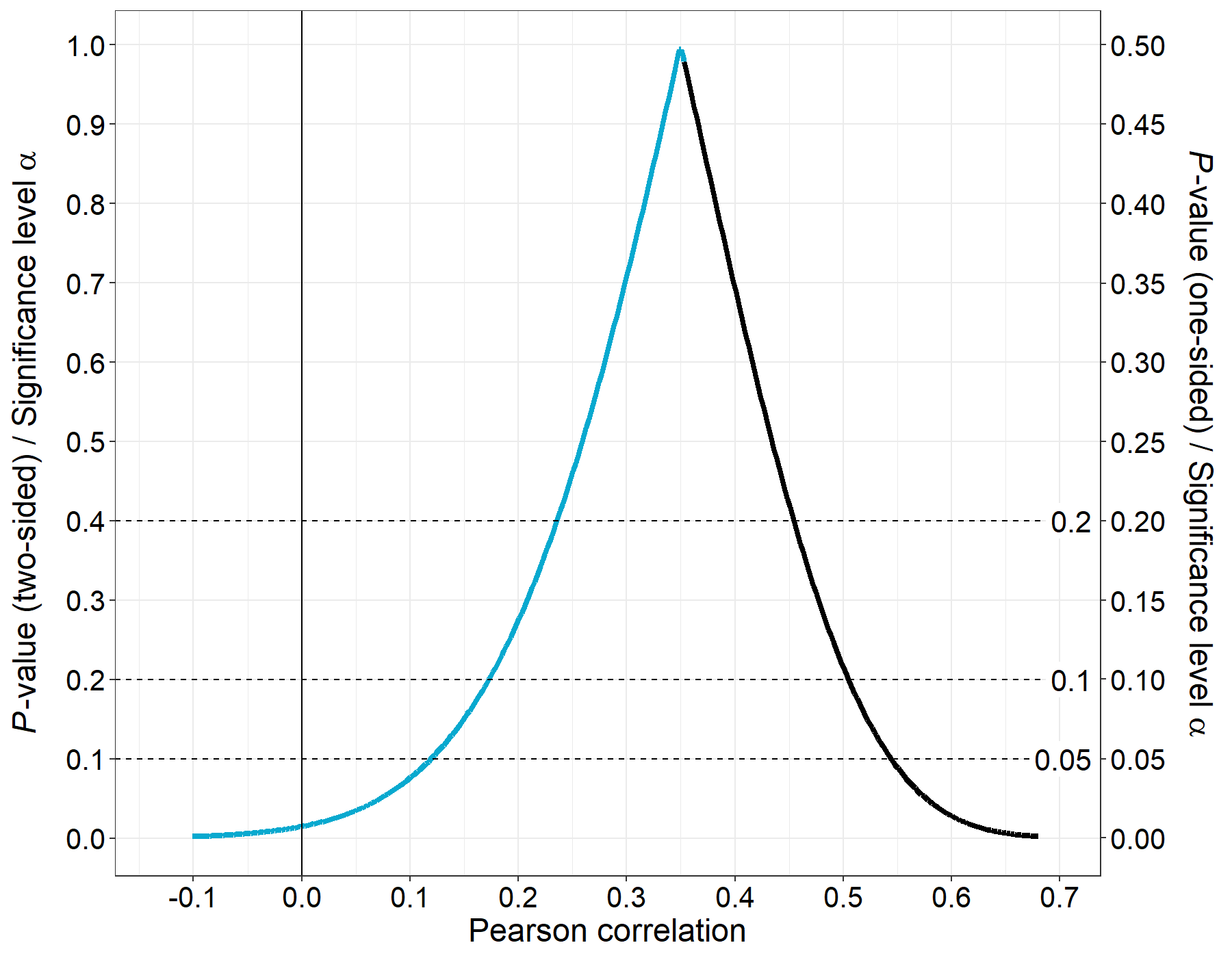Odds ratio from logistic regression

#-----------------------------------------------------------------------------
# Create p-value function
#-----------------------------------------------------------------------------

res <- conf_dist(
estimate = c(0.804037549)
, stderr = c(0.331819298)
, type = "logreg"
, plot_type = "p_val"
, n_values = 1e4L
, est_names = c("GPA")
, conf_level = c(0.95, 0.90, 0.80)
, null_values = c(log(1)) # null value on the log-odds scale
, trans = "exp"
, alternative = "two_sided"
, log_yaxis = FALSE
, cut_logyaxis = 0.05
, xlab = "Odds Ratio (GPA)"
, xlim = log(c(0.7, 5.2)) # axis limits on the log-odds scale
, together = FALSE
, plot_p_limit = 1 - 0.999
, plot_counternull = TRUE
, x_scale = "default"
)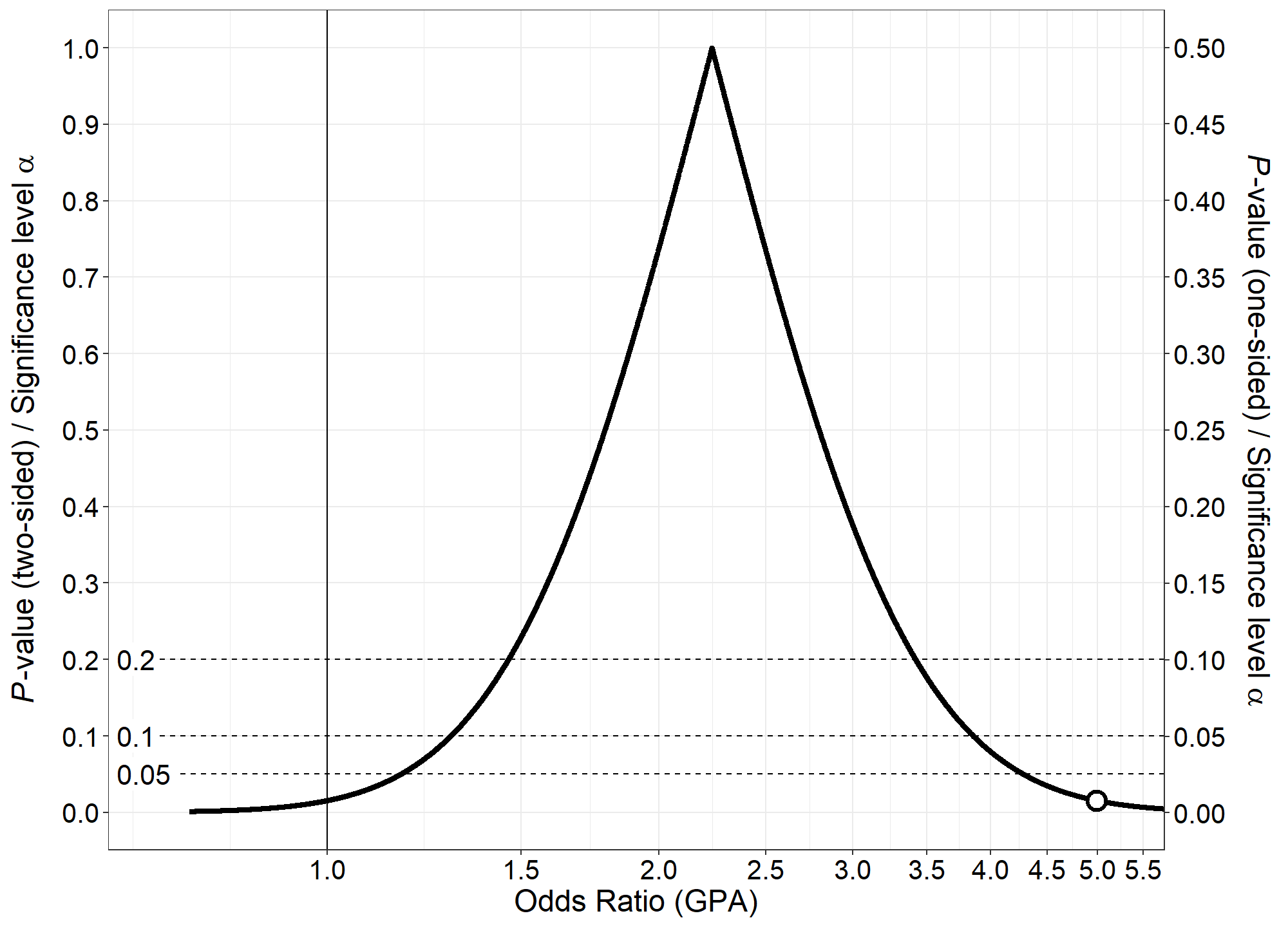Proportion

The p-value function (and thus the confidence intervals) are based on Wilson’s score interval and not the normal approximation. This means that the p-value function will never be outside the interval \([0, 1]\).

res <- conf_dist(
estimate = c(0.44)
, n = c(50)
, type = "prop"
, plot_type = "p_val"
, n_values = 1e4L
# , est_names = c("")
, conf_level = c(0.95, 0.90, 0.80)
, null_values = c(0.5)
, trans = "exp"
, alternative = "two_sided"
, log_yaxis = FALSE
, cut_logyaxis = 0.05
, xlab = "Proportion"
# , xlim = c(0.25, 0.65)
, together = FALSE
, plot_p_limit = 1 - 0.999
, plot_counternull = TRUE
, x_scale = "default"
)
#>
#> Transformation changed to identity.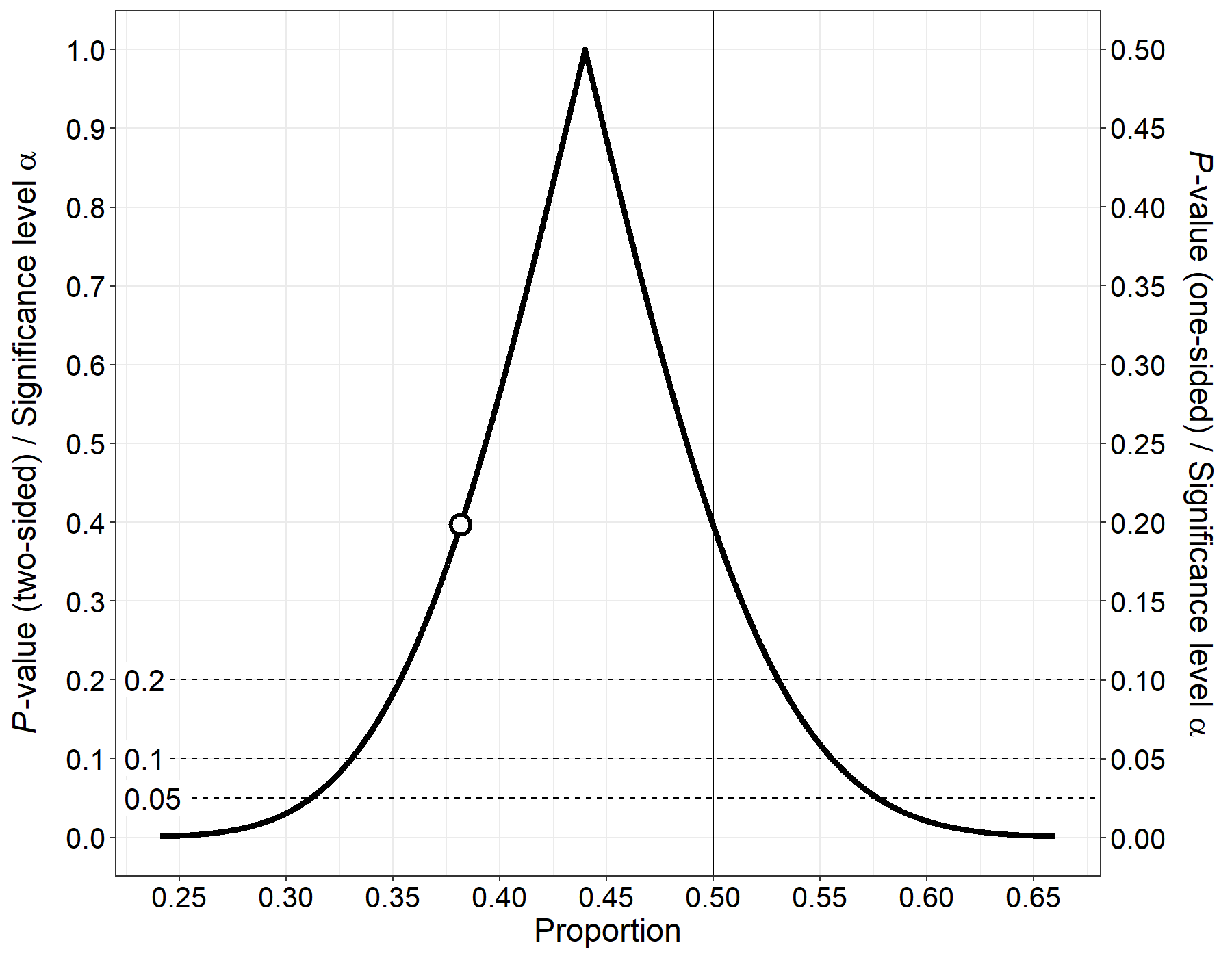Difference between two independent proportions: Wilson’s score by Newcombe with continuity correction

res <- conf_dist(
estimate = c(68/100, 98/150)
, n = c(100, 150)
, type = "propdiff"
, plot_type = "p_val"
, n_values = 1e4L
, conf_level = c(0.95, 0.90, 0.80)
, null_values = c(0)
, trans = "identity"
, alternative = "two_sided"
, log_yaxis = FALSE
, cut_logyaxis = 0.05
, xlab = "Difference between proportions"
, together = FALSE
, plot_p_limit = 1 - 0.9999
, plot_counternull = FALSE
)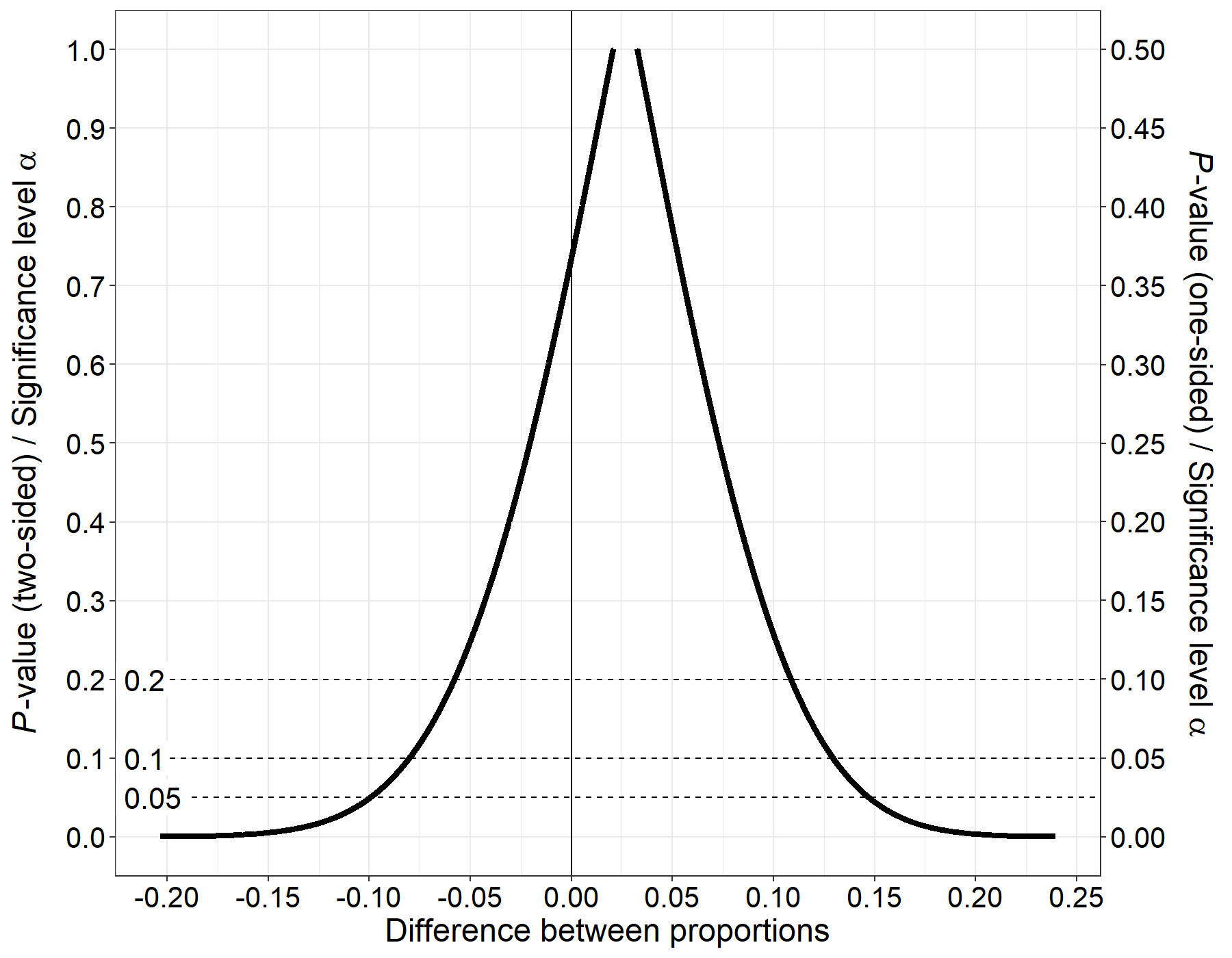Difference between two independent proportions: Agresti-Caffo adjusted Wald interval

The standard Wald interval can be modified in a simple manner to drastically improve its coverage probabilities. Simply add 1 to the number of successes and add 2 to the sample size for both proportions. Then proceed to calculate the Wald interval with these modified data. The point estimate for the difference between proportions is still calculated using the unmodified data. The function conf_dist does not have a dedicaded type for this kind of estimator but as the Wald interval is based on the normal distribution, we can use type = general_z to create the p-value function.

# First proportion

x1 <- 8
n1 <- 40

# Second proportion

x2 <- 11
n2 <- 30

# Apply the correction

p1hat <- (x1 + 1)/(n1 + 2)
p2hat <- (x2 + 1)/(n2 + 2)

# The estimator (unmodified)

est0 <- (x1/n1) - (x2/n2)

# The modified estimator and its standard error using the correction

est <- p1hat - p2hat
se <- sqrt(((p1hat*(1 - p1hat))/(n1 + 2)) + ((p2hat*(1 - p2hat))/(n2 + 2)))

res <- conf_dist(
estimate = c(est)
, stderr = c(se)
, type = "general_z"
, plot_type = "p_val"
, n_values = 1e4L
# , est_names = c("Estimate")
, log_yaxis = FALSE
, cut_logyaxis = 0.05
, conf_level = c(0.95, 0.99)
, null_values = c(0)
, trans = "identity"
, alternative = "two_sided"
, xlab = "Difference of proportions"
# , xlim = c(-0.75, 0.5)
, together = FALSE
, plot_p_limit = 1 - 0.9999
, plot_counternull = FALSE
)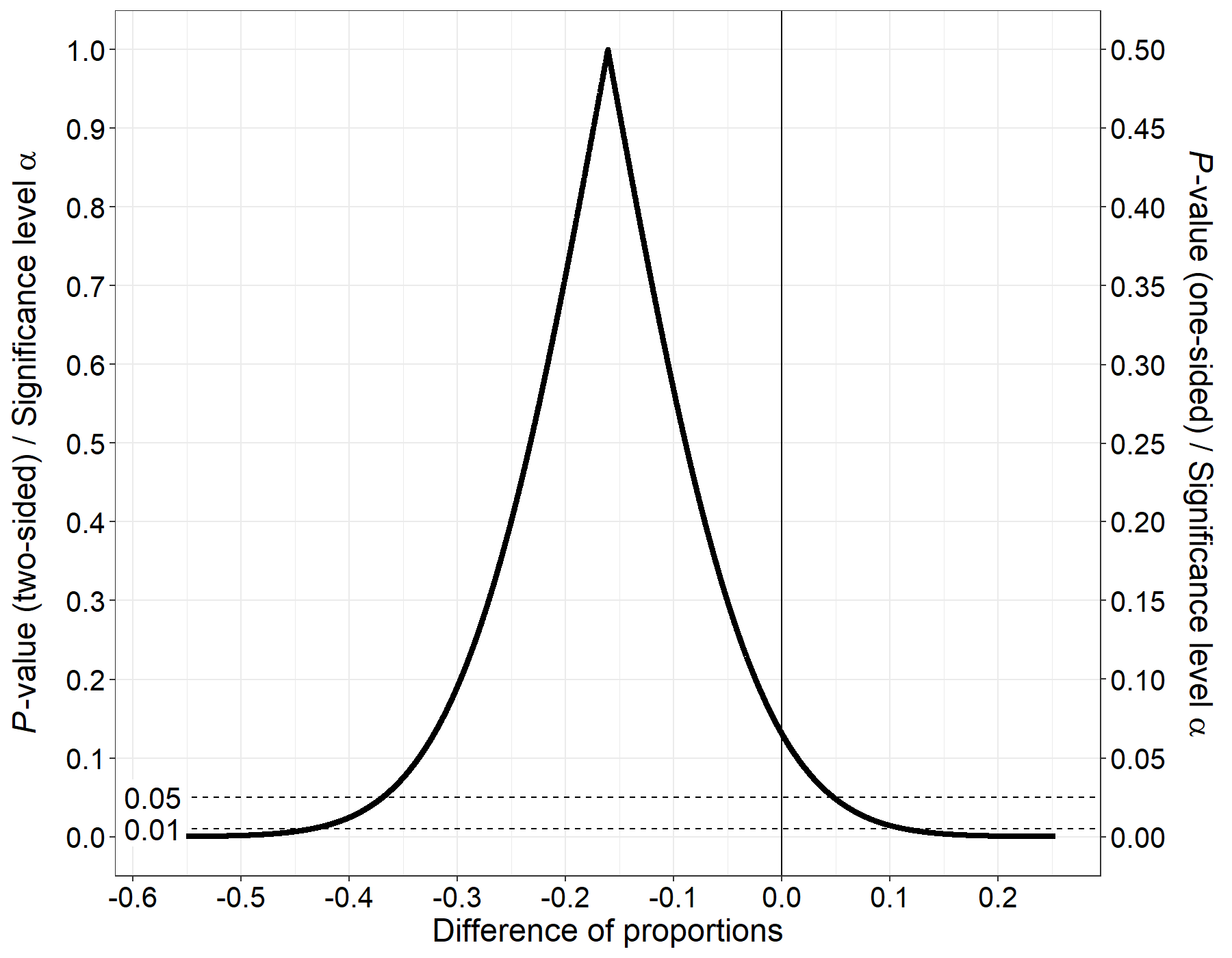Confidence density of a variance estimate from a normal distribution

The confidence density of a variance estimate is skewed. This means that the mean, mode and median of the confidence density will not be identical, in general.

# Simulate some data from a normal distribution

set.seed(142857)
var_est <- var(x <- rnorm(20, 100, 15))

res <- conf_dist(
estimate = var_est
, n = length(x)
, type = "var"
, plot_type = "pdf"
, n_values = 1e4L
, est_names = c("Variance")
, log_yaxis = FALSE
, cut_logyaxis = 0.05
, conf_level = c(0.95)
# , null_values = c(15^2, 18^2)
, trans = "identity"
, alternative = "two_sided"
, xlab = "Variance"
, xlim = c(100, 900)
, together = TRUE
, plot_p_limit = 1 - 0.999
, plot_counternull = TRUE
)
# Add vertical lines at the point estimates (mode, median, mean)

res\$plot + ggplot2::geom_vline(xintercept = as.numeric(res\$point_est[1, 1:3]), linetype = 2, size = 1)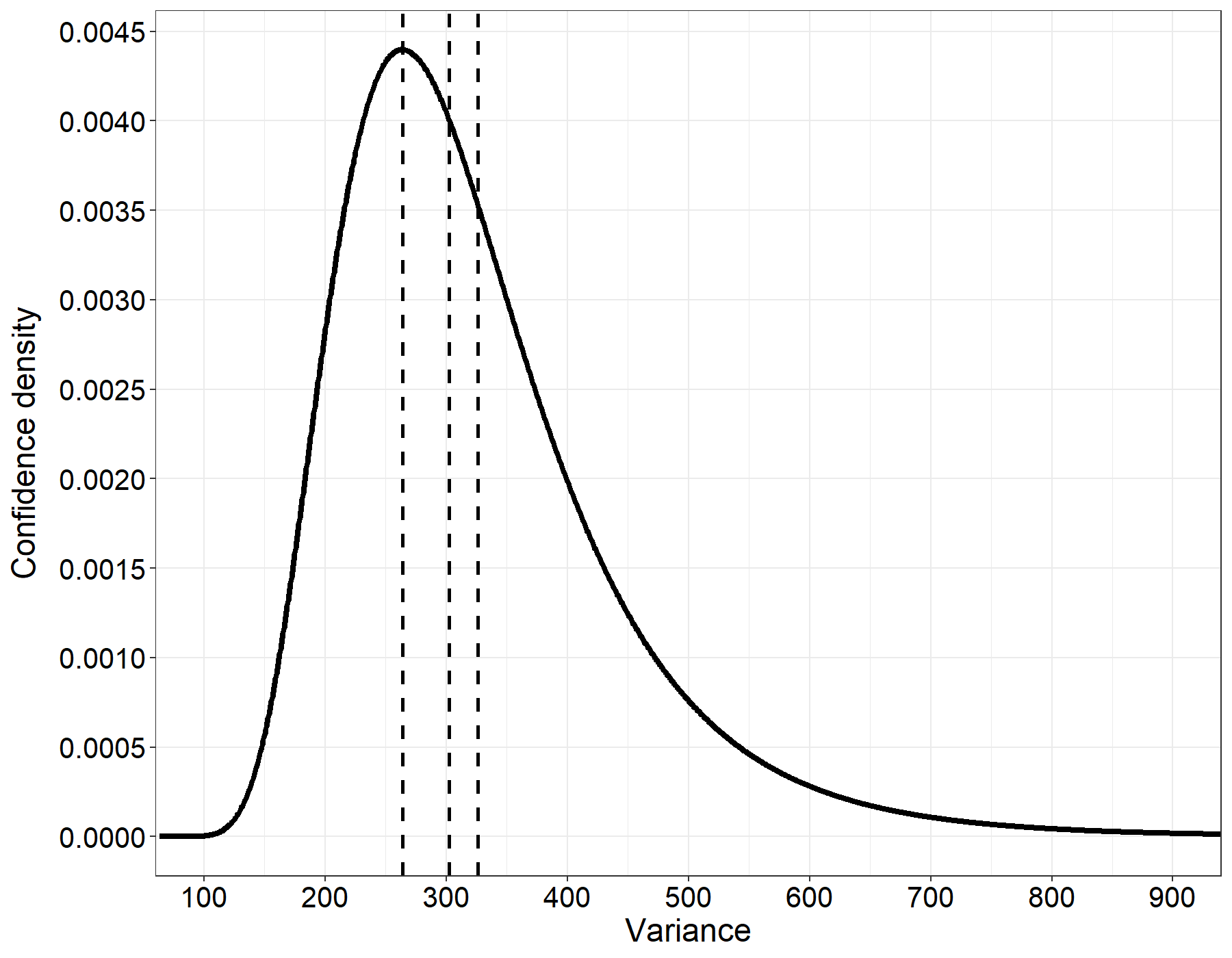P-value function and confidence distribution for the relative survival effect (1 - HR%)

Here, I’m going to replicate Figure 1 and Figure 2 in Bender et al. (2005). To do this, we first need to define the transformation that transforms the log-HR into the relative survival effect. By specifying trans = rse_fun, the values are automatically transformed and plotted. In addition, we will plot the p-value function inverted so that the cusp is located at the bottom. First the p-value function:

# Define the transformation function and its inverse for the relative survival effect

rse_fun <- function(x){
100*(1 - exp(x))
}

rse_fun_inv <- function(x){
log(1 - (x/100))
}

res <- conf_dist(
estimate = c(log(0.72))
, stderr = (0.187618)
, type = "coxreg"
, plot_type = "p_val"
, n_values = 1e4L
, est_names = c("RSE")
, conf_level = c(0.95, 0.8, 0.5)
, null_values = rse_fun_inv(c(0))
, trans = "rse_fun"
, alternative = "two_sided"
, log_yaxis = FALSE
, cut_logyaxis = 0.05
, xlab = "Relative survival effect (1 - HR%)"
, xlim = rse_fun_inv(c(-30, 60))
, together = FALSE
, plot_p_limit = 1 - 0.999
, plot_counternull = TRUE
, inverted = TRUE
, title = "Figure 1 in Bender et al. (2005)"
, x_scale = "default"
)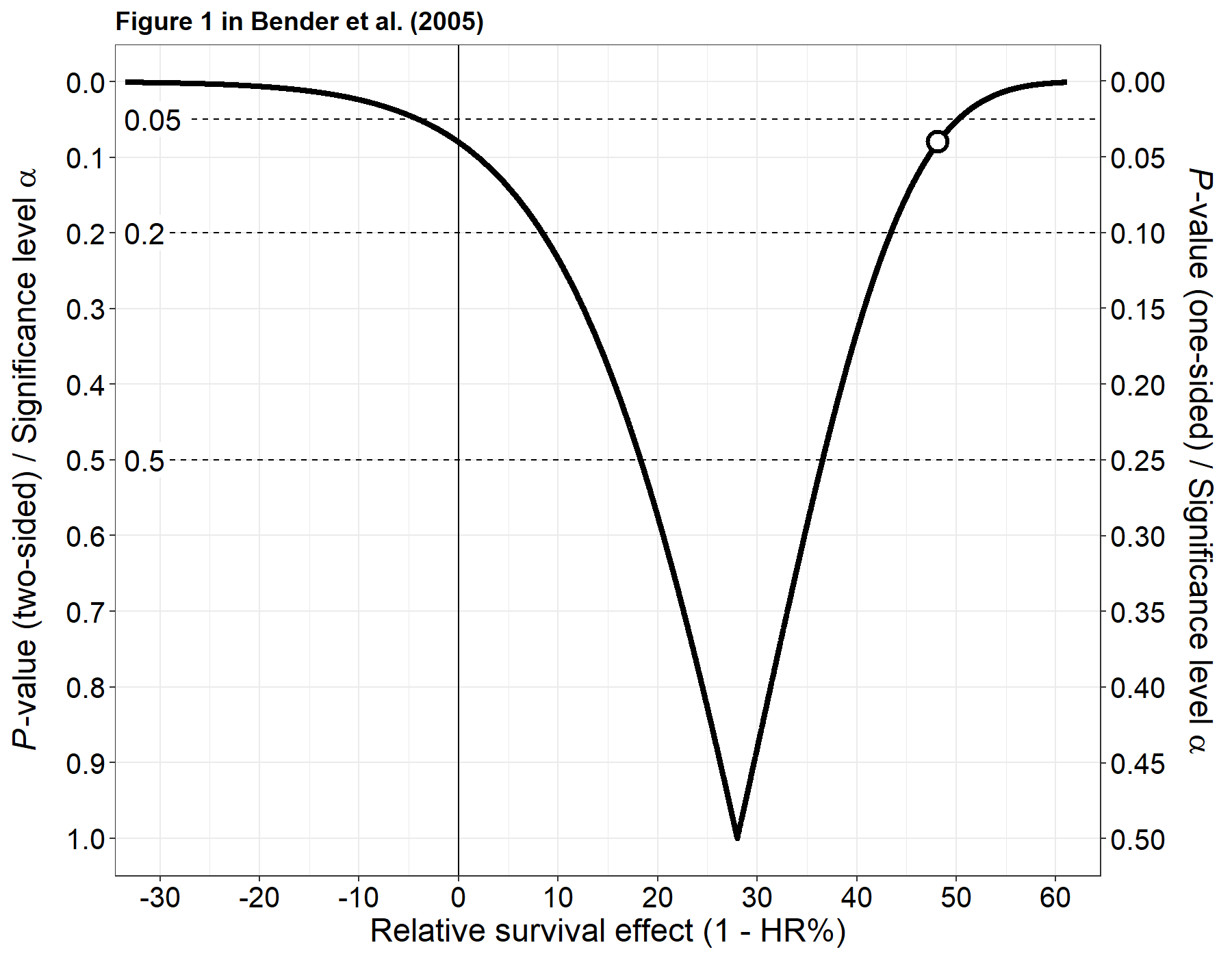rm(rse_fun, rse_fun_inv)

Now the confidence distribution (Figure 2):

# Define the transformation function and its inverse for the relative survival effect

rse_fun <- function(x){
100*(1 - exp(-x))
}

rse_fun_inv <- function(x){
log(-(100)/(x - 100))
}

res <- conf_dist(
estimate = c(-log(0.72))
, stderr = (0.187618)
, type = "coxreg"
, plot_type = "cdf"
, n_values = 1e4L
, est_names = c("RSE")
, conf_level = c(0.95, 0.883, 0.5)
, null_values = rse_fun_inv(c(0))
, trans = "rse_fun"
, alternative = "two_sided"
, log_yaxis = FALSE
, cut_logyaxis = 0.05
, xlab = "Relative survival effect (1 - HR%)"
, together = FALSE
, xlim = rse_fun_inv(c(-3, 60))
, plot_p_limit = 1 - 0.999
, plot_counternull = TRUE
, inverted = TRUE
, title = "Figure 2 in Bender et al. (2005)"
)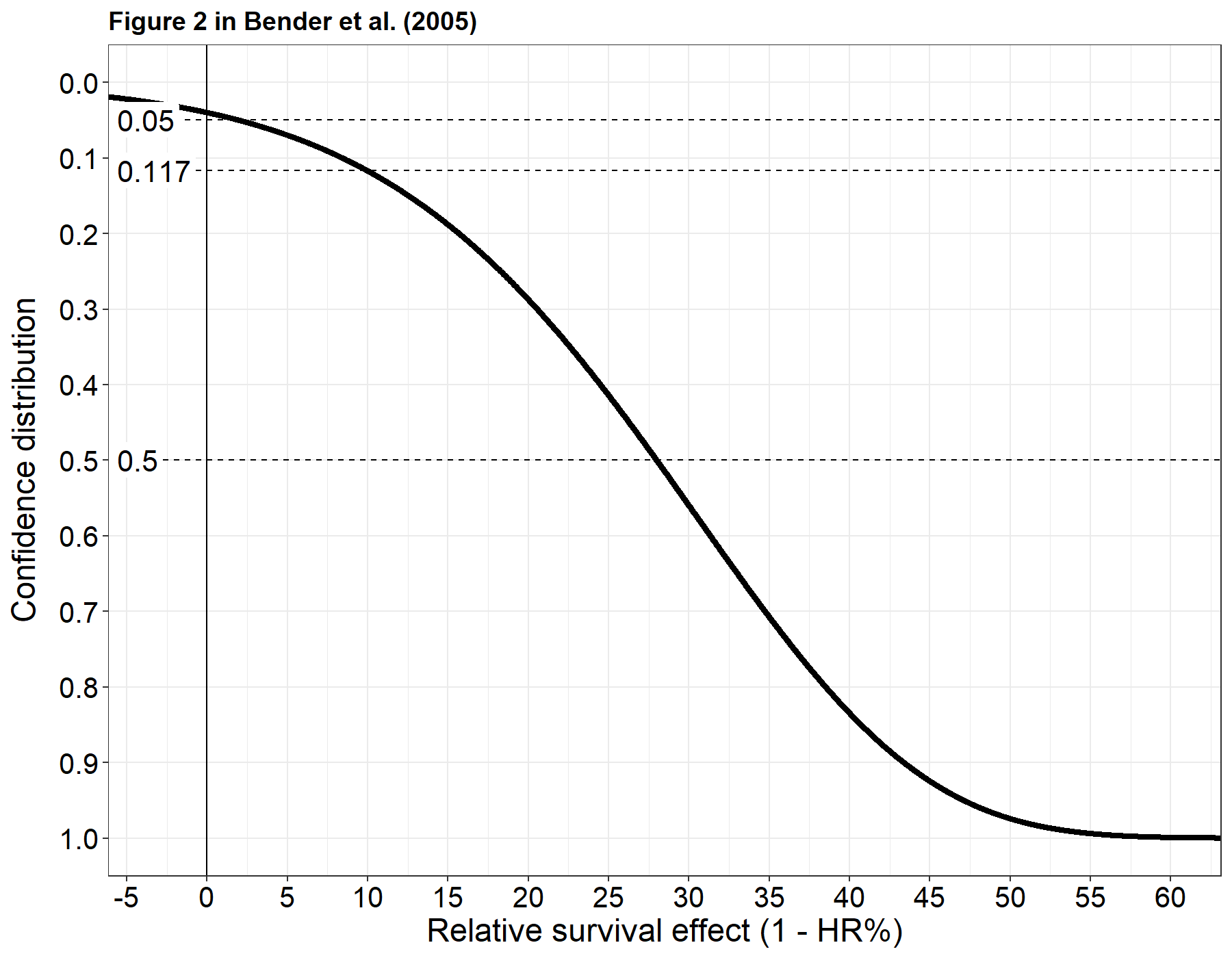rm(rse_fun, rse_fun_inv)

Comparing the precision of multiple estimates using the AUCC (area under the confidence curve)

The AUCC is a scalar representing the area under the p-value function (see Berrar (2017)). Values close to 0 indicate a high precision w